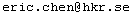# Two-Weight Codes

## 1.  Projective Two-Weight Codes

A linear code is called projective if any two of its coordinates are linearly independent, or in other words, if the minimum distance of its dual code is at least three. A code is called the two-weight code if any nonzero codeword has a weight w1 or w2.

Follow the link to search the online database of two-weight codes. You are welcome to send me your constructions of two-weight codes (  eric.chen at hkr.se).

## 2. Strongly Regular Graphs

A strongly regular graph with parameters (N, K, λ, μ) is a finite simple graph on N vertices, regular of degree K, and such that any two distinct vertices have λ common neighbours when they are adjacent and  μ  common neighbours when they are not adjacent. A projective two-weight code specifies a strongly regular graph.

A q-ary projective two-weight code specifies a strongly regular graph. Let w
1 and w2(we suppose  w1 < w2) be two weights of a projective q-ary two-weight [n, k] code. A strongly regular graph can be constructed as follows. The vertices of the graph are identified by the codewords, and two vertices x and y are adjacent if and only d(x, y) = w1. The resulting strongly regular graph has parameters (N, K, λ, μ ) given by :

N = qk,

K = n (q-1)

λ =  K2 + 3K –q(w1 + w2) – Kq(w1 + w2) + q2 w1w2

μ = q2 w1w2 / qk = K2 + K –Kq(w1 + w2) + q2 w1w2

 R. CALDERBANK AND W. M. KANTOR, "THE GEOMETRY OF TWO-WEIGHT CODES", Bull. London Math. Soc. 18 (1986) 97-122